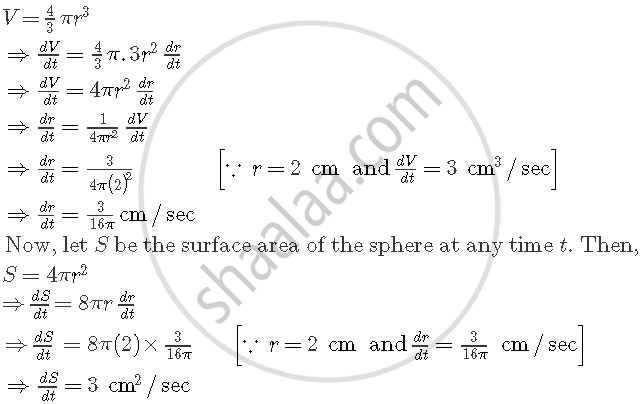Share

# The Volume of a Sphere is Increasing at the Rate of 3 Cubic Centimeter per Second. Find the Rate of Increase of Its Surface Area, When the Radius is 2 Cm - CBSE (Commerce) Class 12 - Mathematics

ConceptRate of Change of Bodies Or Quantities

#### Question

The volume of a sphere is increasing at the rate of 3 cubic centimeter per second. Find the rate of increase of its surface area, when the radius is 2 cm

#### Solution

Let r be the radius and V be the volume of the sphere at any time t. Then,Is there an error in this question or solution?

#### Video TutorialsVIEW ALL 

Solution The Volume of a Sphere is Increasing at the Rate of 3 Cubic Centimeter per Second. Find the Rate of Increase of Its Surface Area, When the Radius is 2 Cm Concept: Rate of Change of Bodies Or Quantities.
S